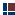The Temple and 1260 days of Bible Prophecy

Measuring the Tabernacle Curtains
and comparing them with Bible-Prophecy time spans

Continued

1320 and 1260 sq. cubits Discussed

(Based on Exodus 26)

Meaning of curtains as 1320 square cubits:

Before we look at the more striking figure of '1260 square cubits' for the curtains, (for that is what they can also add up to), let us quickly look at the meaning of the '1320.'

The measurement of '1320' is an interesting number in the study of the 360 bible calendar. For example, let us say that 1320 square cubits symbolically represents 1320 years. The total of all 4 curtain material is thus 1320 x 4 = 5280 symbolic 'years'

Now, every 480 years in the 360 calendar accumulates exactly 84 leap months, (which is 1260 x 2 days, or, 7 years of 360 days). A seven-year period is an important length of time in the bible, (Revelation 11-12.)

---Every 40 years accumulates 7 leap-months (210 days).
and 40 years times 12 = 480 years.
---Therefore, there are 84 leap months (2520 days) per 480-year period.

The total said figure of 5280 (square cubits) as years can be divided into 11 480-year units. (480 x 11 = 5280). Therefore, every 5280 years accumulates 77 years of leap months.

---7 years per 480 years, x 11 cycles of 480 = 77 years

The number '77' continues the theme of the 7’s, and in the context of the Tabernacle reminds us of the message of forgiveness. Jesus said: "How often shall I forgive my brother, till 77 times (or 70 x 7)?" This, in turn, agrees with the general meaning of the curtain coverings, that is, "a covering (forgiveness) for sins by sacrifice."

Meaning of curtains as 1260 square cubits:

(Note the area of the curtain is 30 by 4: There are '30' days in a month, and '4' seasons in a year.)

The first two "tent curtains," therefore, can be said to be 1320 + 1260 (2640) square cubits. This yields an average of 1290 square feet per curtain. (1290 x 2 = 2640, Daniel 12:11, and is 430 x 6 (= 2640) of Ezekiel 4:4-10, as well.)

Hence, the first two curtains (which the bible itself groups together) are called, "tent curtains" and therefore symbolize one seven-year period (2 x 3.5 years), whereas the outer two coverings (called simply "coverings") likewise represent a divided seven year period, (just as the bible divides it, Daniel 9:24-27; 12:11, Revelation 11: 2-3; Rev 12:6).

The fact that the 1120 of the first covering of fine linen (without the 200 square cubits for the two entrances) is also 1260 x 2 square feet (as you said) probably implies in Bible Prophecy that the Gentiles would be graciously ushered into the very presence of God, since units of feet are Gentile in origin, and likely trace back to ancient Babylon. It might also imply "the abomination of desolation" (Matt. 24; cf., Dan 9:24-27) of 1260 days since the material that covered the Holy of Holies was thus 1260 square feet.

Also see, How Israel became a nation in 1948 on the exact date predicted in the Bible,
1260 x 2 years from Ezekiel's temple!

==================

Bible Prophecy Numbers

 Begin here! Bible Prophecy NumbersIntroduction  (Writing on the Wall) Intro. a   A Secret Message Intro. b   Purpose and ScopeChapter One  ("Yes It is I") Ch. 1a   Ten Epoch Events  (Detailed)  Ch. 1b   Seven-Year Famines Ch. 1c   What Prophecy Numbers RevealChapter Two ("How Long?")
Ch. 2a   General Meaning of "How Long?"
Ch. 2b   The Famine of Joseph
Ch. 2c   A-Day-Equals-a-Year
Ch. 2d   What are the Bible Prophecy Numbers?
Ch. 2e   390, 430, (1290), and the 1150
Ch. 2f    1260 days/years, 1290 days/years
Ch. 2g   3½-years Bible-Prophecy, Solar, Lunar  (Detailed
Ch. 2h   T
ime, Times, and a Half-a-time (Detailed
Ch. 2i    1335 of Daniel and 430 of Ezekiel
Ch. 2j     (Endnotes 1-11)  and 2k  (Endnotes 12-23)Chapter Three ("The Mirror")In Chapter Four we discuss the principle that "Each day equals a consecutive year/date of time".    Still in rough form.Chapter Five, Jubilee & Grand Jubilee Cycles   (In rough)